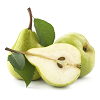#### You may also like### Seven Squares - Group-worthy Task

Choose a couple of the sequences. Try to picture how to make the next, and the next, and the next... Can you describe your reasoning?### Pair Products Poster

Pair Products Poster### Committees

Every member of the club is a member of two committees. How many members are there in the club?

# Marbles in A Box Poster

##### Age 11 to 14Challenge Level

There are 49 winning lines.

Click below to see four different methods.

Alison's method

There are $3$ possible places where a line can start:
• at a vertex
• at the middle of an edge
• in the centre of a face
A cube has $8$ vertices, $12$ edges and $6$ faces.

From a vertex there are $7$ other vertices that you can join to in order to make a winning line.  $7 \times 8 = 56$ lines, but this counts each line from both ends, so there are $28$ 'vertex' winning lines.

From the middle of an edge there are $3$ other middles-of-edges that you can join to in order to make a winning line.  $3 \times 12 = 36$ lines, but this counts each line from both ends so there are $18$ 'middle of edge' winning lines.

From the centre of each face there is one winning line, joining to the opposite face, so there are $3$ 'centre of face' winning lines.

So in total, there are $28 + 18 + 3 = 49$ winning lines.

James' method

Winning lines can either be:
• Diagonal
• Not diagonal
Considering the non-diagonal winning lines first:
There are $9$ from front to back.
There are $9$ from left to right.
There are $9$ from top to bottom.

Considering the diagonal winning lines:

On each layer there are $2$ diagonal winning lines so:
There are $6$ from front to back.
There are $6$ from top to bottom.
There are $6$ from left to right.

There are $4$ lines from a vertex to a diagonally opposite vertex.

In total, there are $27+18+4=49$ winning lines.

Caroline's method

All winning lines must pass either:
• along an edge of the cube
• through the middle of a face
• through the centre of the cube

There are $12$ edges on a cube so there are $12$ winning lines along edges.

There are $6$ faces on a cube, and $4$ winning lines that pass through the middle of each face, so there are $24$ winning lines through the middle of faces.

Finally we need to consider the winning lines that go through the centre cube:

vertex to opposite vertex: $4$
middle of edge to middle of opposite edge: $6$
middle of face to middle of opposite face: $3$

In total, there are $12 + 24 + 4 + 6 + 3 = 49$ winning lines.

Grae's method

The winning lines may be counted by looking at lines:
• in each horizontal plane
• in each vertical plane from left to right
• in each vertical plane from front to back
• in the diagonal planes

On a plane there are $8$ winning lines.
In the cube, there are $3$ horizontal planes, so $8 \times 3 = 24$ winning lines.

There are also $3$ vertical planes going from left to right, but now with only $5$ new winning lines per plane, as the $3$ horizontal lines have already been counted.  So $5 \times 3 = 15$ winning lines.

On the $3$ vertical planes going from front to back, we now only have $2$ new (diagonal) winning lines per plane. So $2 \times 3 = 6$ winning lines.

Finally, there are also diagonal planes to consider. There are $4$ winning lines going from corner to diagonally opposite corner.

In total, there are $24 + 15 + 6 + 4 = 49$ winning lines.